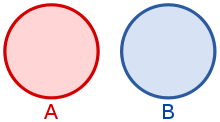# Disjoint sets

Disjoint setsTwo disjoint sets.

In mathematics, two sets are said to be disjoint if they have no element in common. For example, {1, 2, 3} and {4, 5, 6} are disjoint sets.

## Explanation

Formally, two sets A and B are disjoint if their intersection is the empty set, i.e. if$A\cap B = \varnothing.\,$

This definition extends to any collection of sets. A collection of sets is pairwise disjoint or mutually disjoint if, given any two sets in the collection, those two sets are disjoint.

Formally, let I be an index set, and for each i in I, let Ai be a set. Then the family of sets {Ai : iI} is pairwise disjoint if for any i and j in I with ij,$A_i \cap A_j = \varnothing.\,$

For example, the collection of sets { {1}, {2}, {3}, ... } is pairwise disjoint. If {Ai} is a pairwise disjoint collection (containing at least two sets), then clearly its intersection is empty:$\bigcap_{i\in I} A_i = \varnothing.\,$

However, the converse is not true: the intersection of the collection {{1, 2}, {2, 3}, {3, 1}} is empty, but the collection is not pairwise disjoint. In fact, there are no two disjoint sets in this collection.

A partition of a set X is any collection of non-empty subsets {Ai : iI} of X such that {Ai} are pairwise disjoint and$\bigcup_{i\in I} A_i = X.\,$

Wikimedia Foundation. 2010.

### Look at other dictionaries:

• Almost disjoint sets — In mathematics, two sets are almost disjoint if their intersection is small in some sense. Different definitions of small will therefore result in different definitions of almost disjoint . Definition The most common choice is to take small to… …   Wikipedia

• Disjoint — may refer to: Disjoint sets Disjoint union This disambiguation page lists articles associated with the same title. If an internal link led you here, you may wish to change the link to point directly to the intende …   Wikipedia

• Disjoint union — In mathematics, the term disjoint union may refer to one of two different concepts: In set theory, a disjoint union (or discriminated union) is a modified union operation that indexes the elements according to which set they originated in;… …   Wikipedia

• Disjoint-set data structure — In computing, a disjoint set data structure is a data structure that keeps track of a set of elements partitioned into a number of disjoint (nonoverlapping) subsets. A union find algorithm is an algorithm that performs two useful operations on… …   Wikipedia

• disjoint — [dis joint′, dis′joint΄] adj. [ME < OFr desjoint, pp. of desjoindre: see DISJOIN] 1. Obs. disjointed 2. Math. of sets having no members in common vt. [< DISJOINT the adj.] 1. to put out of joint; dislocate …   English World dictionary

• Disjoint union (topology) — In general topology and related areas of mathematics, the disjoint union (also called the direct sum, free union, free sum, topological sum, or coproduct) of a family of topological spaces is a space formed by equipping the disjoint union of the… …   Wikipedia

• disjoint — /dis joynt /, v.t. 1. to separate or disconnect the joints or joinings of. 2. to put out of order; derange. v.i. 3. to come apart. 4. to be dislocated; be out of joint. adj. 5. Math. a. (of two sets) having no common elements. b. (of a system of… …   Universalium

• disjoint — dis•joint [[t]dɪsˈdʒɔɪnt[/t]] v. t. 1) to separate or disconnect the joints or joinings of 2) to put out of order; derange 3) to come apart 4) to be dislocated; be out of joint 5) math. Math. a) (of two sets) having no common elements b) (of a… …   From formal English to slang

• disjoint — 1. adjective a) not smooth or continuous; disjointed b) (not used in the comparative or superlative) Of two or more sets, having no members in common; having an intersection equal to the empty set. Ant: non disjoint …   Wiktionary

• disjoint — I. adjective Etymology: Middle English disjoynt, from Anglo French desjoint, past participle of desjoindre Date: 15th century 1. obsolete disjointed 1a 2. having no elements in common < disjoint mathematical sets > II …   New Collegiate Dictionary